In :
%matplotlib inline
from IPython.display import Image
from IPython.html.widgets import interact

In :
Image('Lighthouse_schematic.jpg',width=500)

Out: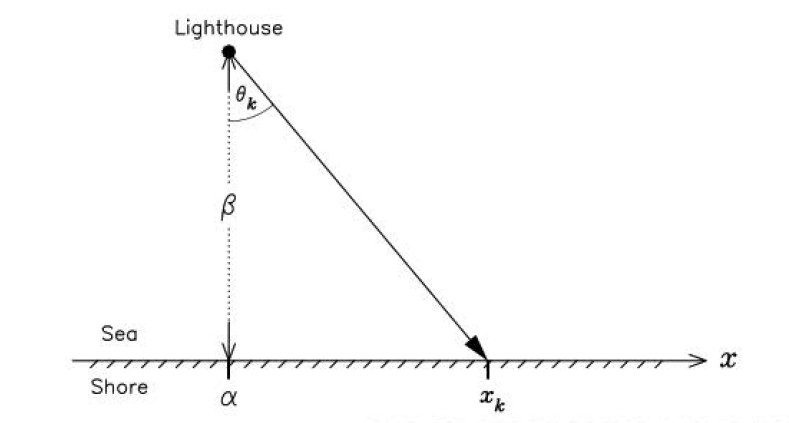The following is a classic estimation problem called the "lighthouse problem". The figure shows a set of receivers distributed at coordinates $x_k$ along the shore and a lighthouse located at some position $(\alpha,\beta)$ offshore. The idea is that the coastline is equipped with a continuous strip of photodetectors. The lighthouse flashes the shore $N$ times at some random angle $\theta_k$. The strip of photodetectors registers the $k^{th}$ flash position $x_k$, but the the angle $\theta_k$ of the flash is unknown. Furthermore, the lighthouse beam is laser-like so there is no smearing along the strip of photodetectors. In other words, the lighthouse is actually more of a disco-ball in a dark nightclub.

The problem is how to estimate $\alpha$ given we already have $\beta$.

From basic trigonometry, we have the following:

$$\beta \tan(\theta_k) = x_k - \alpha$$

The density function for the angle is assumed to be the following:

$$f_{\alpha}(\theta_k) = \frac{1}{\pi}$$

This means that the density of the angle is uniformly distributed between $\pm \pi/2$. Now, what we really want is the density function for $x_k$ which will tell us the probability that the $k^{th}$ flash will be recorded at position $x_k$. After a transformation of variables, we obtain the following:

$$f_{\alpha}(x_k) = \frac{\beta}{\pi(\beta ^2 +(x_k-\alpha)^2)}$$

which we plot below for some reasonable factors

In :
xi = linspace(-10,10,150)
alpha = 1
f = lambda x: 1/(pi*(1+(x-alpha)**2))
plot(xi,f(xi))
xlabel('$x$',fontsize=24)
ylabel(r'$f_{\alpha}(x)$',fontsize=24);
vlines(alpha,0,.35,linestyles='--',lw=4.)
grid()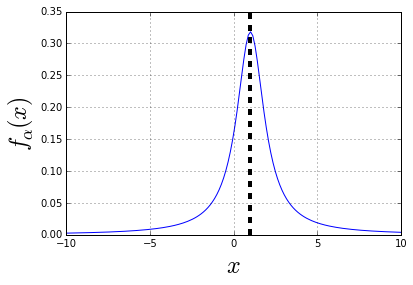As shown in figure, the peak for this distribution is when $x_k=\alpha$. Because there is no coherent processing, the recording of a signal at one position does not influence what we can infer about the position of another measurement. Thus, we can justify assuming independence between the individual $x_k$ measurements. The encouraging thing about this distribution is that it is centered at the variable we are trying to estimate, namely $\alpha$.

The temptation here is to just average out the $x_k$ measurements because of this tendency of the distribution around $\alpha$. Later, we will see why this is a bad idea.

## Using Maximum Likelihood Estimation¶

Given $N$ measurements, we form the likelihood as the following:

$$L(\alpha) = \prod_{k=1}^N f_{\alpha}(x_k)$$

And this is the function we want to maximize for our maximum likelihood estimator. Let's process the logarithm of this to make the product easy to work with as this does not influence the position of the maximum $\alpha$. Then,

$$\mathcal{L}(\alpha)=\sum_{k=1}^N \ln f_{\alpha}(x_k) = \texttt{constant}- \sum_{k=1}^N \ln (\beta ^2 +(x_k-\alpha)^2)$$

Taking the first derivative gives us the equation would have to solve in order to compute the estimator for $\alpha$,

$$\frac{d \mathcal{L}}{d \alpha} = 2 \sum_{k=1}^N \frac{x_k-\alpha}{\beta^2+(x_k-\alpha)^2}=0$$

Unfortunately, there is no easy way to solve for the optimal $\alpha$ for this equation. However, we are not defenseless at this point because Python has all the tools we need to overcome this. Let's start by getting a quick look at the histogram of the $x_k$ measurements.

In :
beta  =alpha = 1
theta_samples=((2*np.random.rand(250)-1)*pi/2)
x_samples = alpha+beta*np.tan(theta_samples)
hist(x_samples);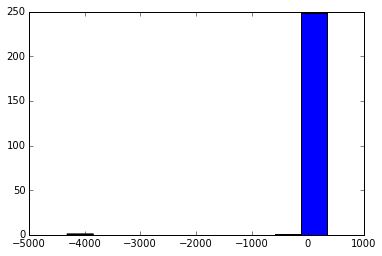The histogram above shows that although many of the measurements are in one bin, even for relatively few samples, we still observe measurements that are very for displaced from the main group. This is because we initially assumed that the $\theta_k$ angle could be anywhere between $[-\pi/2,\pi/2]$, which is basically expressing our ignorance of the length of the coastline.

With that in mind, let's turn to the maximum likelihood estimation. We will need some tools from sympy.

In :
import sympy as S
a=S.symbols('alpha',real=True)
x = S.symbols('x',real=True)
# form derivative of the log-likelihood
dL=sum((xk-a)/(1+(xk-a)**2)  for xk in x_samples)
S.plot(dL,(a,-15,15),xlabel=r'$\alpha$',ylabel=r'$dL(\alpha)/d\alpha$')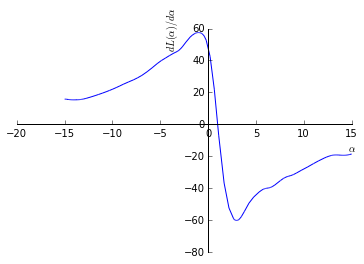Out:
<sympy.plotting.plot.Plot at 0x94adb90>

The above figure shows that the zero-crossing of the derivative of the log-likelihood crosses where the $\alpha$ is to be estimated. Thus, our next task is to solve for the zero-crossing, which will then reveal the maximum likelihood estimate of $\alpha$ given the set of measurements $\lbrace x_k \rbrace$.

There are tools in scipy.optimize that can help us compute the zero-crossing as demonstrated in the cell below.

In :
from scipy import optimize
from scipy.optimize import fmin_l_bfgs_b

# convert sympy function to numpy version with lambdify

print alpha_x

(array([ 0.99435905]), array([  3.76209349e-14]), {'warnflag': 2, 'task': 'ABNORMAL_TERMINATION_IN_LNSRCH', 'grad': array([  1.64970679e-05]), 'nit': 5, 'funcalls': 150})


## Comparing The Maximum likelihood Estimator to Just Plain Averaging¶

Whew. That was a lot of work to compute the maximum likelihood estimation. Earlier we observed that the density function is peaked around the $\alpha$ we are trying to estimate. Why not just take the average of the $\lbrace x_k \rbrace$ and use that to estimate $\alpha$?

Let's try computing the average in the cell below.

In :
print 'alpha using average =',x_samples.mean()
print 'maximum likelihood estimate = ', alpha_x

alpha using average = -14.7935661293
maximum likelihood estimate =  [ 0.99435905]


If you run this notebook a few times, you will see that estimate using the average has enormous variance. This is a consequence of the fact that we can have very large absolute values for $\lbrace x_k \rbrace$ corresponding to values of $\theta_k$ near the edges of the $[-\pi/2,\pi/2]$ interval.

In :
def run_trials(n=100):
o=[]
for i in range(100):
theta_samples=((2*np.random.rand(250)-1)*pi/2)
x_samples = alpha+beta*np.tan(theta_samples)
o.append(x_samples)
return np.array(o)

In :
o= run_trials()


The following figure shows the histogram of the measurements. As shown, there are many measurements away from the central part. This is the cause of our widely varying average. What if we just trimmed away the excess outliers? Would that leave us with an easier to implement procedure for estimating $\alpha$?

In :
hist(o[np.where(abs(o)<200)]);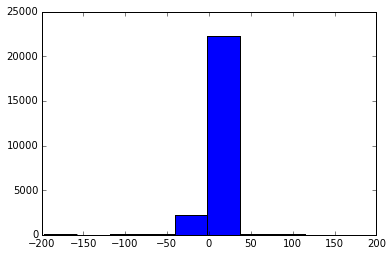The following graph shows what happens when we include only a relative neighborhood around zero in our calculation of the average value. Note that the figure shows a wide spread of average values depending upon how big a neighborhood around zero we decide to keep. This is an indication that the average is not a good estimator for our problem because it is very sensitive to outliers.

In :
plot(range(100,10000,100),[o[np.where(abs(o)<i)].mean() for i in range(100,10000,100)],'-o')
xlabel('width of neighborhood around zero')
ylabel('value of average estimate')

Out:
<matplotlib.text.Text at 0x9a27430>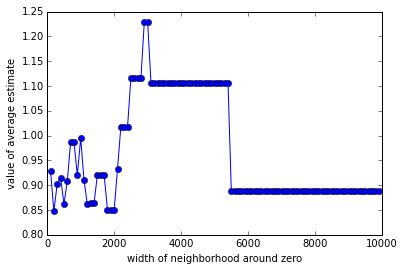For some perspective, we can wrap our maximum likelihood estimator code in one function and then examine the variance of the estimator using our set of synthetic trials data. Note that this takes a long time to run!

In :
def ML_estimator(x_samples):
dL=sum((xk-a)/(1+(xk-a)**2)  for xk in x_samples)
return a_x

In :
# run maximum likelihood estimator on synthetic data we generated earlier
# Beware this may take a long time!
v= np.hstack([ML_estimator(o[i,:]) for i in range(o.shape)])

In :
vmed= np.hstack([np.median(o[i,:]) for i in range(o.shape)])
vavg =  np.hstack([np.mean(o[i,:]) for i in range(o.shape)])
fig,ax = subplots()
ax.plot(v,'-o',label='ML')
ax.plot(vmed,'gs-',label='median')
ax.plot(vavg,'r^-',label='mean')
ax.axis(ymax=2,ymin=0)
ax.legend(loc=(1,0))
ax.set_xlabel('Trial Index')
ax.set_ylabel('Value of Estimator')

Out:
<matplotlib.text.Text at 0x9a2ca30>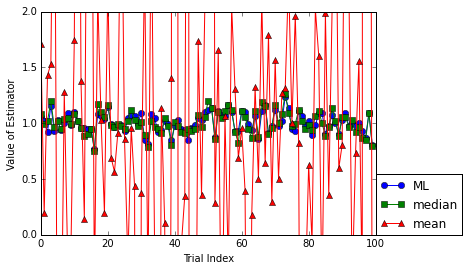The above chart shows that the using the mean-based estimator jumps all over the place while the maximum likelihood (ML) and median-based estimators are less volatile. The next chart explores the relationship between the ML and median-based estimators and checks whether one is biased compared to the other. The figure below shows that (1) there is a small numerical difference between the two estimators (2) neither is systematically different from the other (otherwise, the diagonal would not split them so evenly ).

In :
fig,ax = subplots()
ii= np.argsort(v)
ax.plot(v[ii],vmed[ii],'o',alpha=.3)
axs = ax.axis()
ax.plot(linspace(0,2,10),linspace(0,2,10))
ax.axis(axs)
ax.set_aspect(1)
ax.set_xlabel('ML estimate')
ax.set_ylabel('Median estimate')

Out:
<matplotlib.text.Text at 0x9a45d30>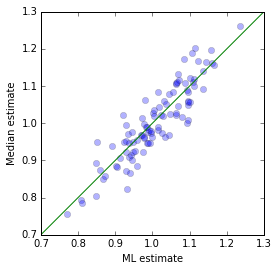In :
fig,ax = subplots()
ax.hist(v,10,alpha=.3,label='ML')
ax.hist(vmed,10,alpha=.3,label='median')
ax.legend(loc=(1,0))

Out:
<matplotlib.legend.Legend at 0x1aa258f0>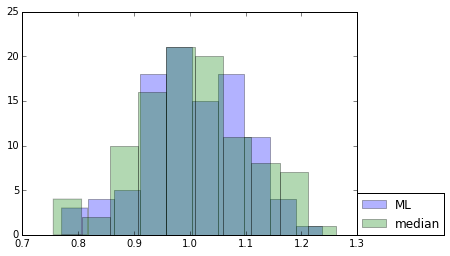## Maximum A-Posteriori (MAP) Estimation¶

Let's use a uniform distribution for the prior of $\alpha$ around some bracketed interval

$$f(\alpha) = \frac{1}{\alpha_{max}-\alpha_{min}} \quad where \quad \alpha_{max} \le \alpha \le \alpha_{min}$$

and zero otherwise. We can compute this sample-by-sample to see how this works using this prior.

In :
alpha  = a
alphamx,alphamn=3,-3
g = f(x_samples)
xi = linspace(alphamn,alphamx,100)
mxval = S.lambdify(a,g)(xi).max()
plot(xi,S.lambdify(a,g)(xi),x_samples,mxval*1.1,'o')

Out:
[<matplotlib.lines.Line2D at 0x1aacd610>,
<matplotlib.lines.Line2D at 0x1aacd770>]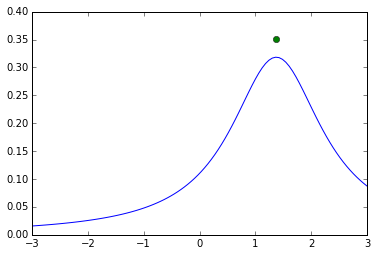In :
xi = linspace(alphamn,alphamx,100)
palpha=S.Piecewise((1,abs(x)<3),(0,True))

def slide_figure(n=0):
fig,ax=subplots()
palpha=S.Piecewise((1,abs(x)<3),(0,True))
if n==0:
ax.plot(xi,[S.lambdify(x,palpha)(i) for i in xi])
ax.plot(x_samples,1.1,'o',ms=10.)
else:
g = S.prod(map(f,x_samples[:n]))
mxval = S.lambdify(a,g)(xi).max()*1.1
ax.plot(xi,S.lambdify(a,g)(xi))
ax.plot(x_samples[:n],mxval*ones(n),'o',color='gray',alpha=0.3)
ax.plot(x_samples[n],mxval,'o',ms=10.)
ax.axis(ymax=mxval*1.1)
ax.set_xlabel(r'$\alpha$',fontsize=18)
ax.axis(xmin=-17,xmax=17)

In :
interact(slide_figure, n=(0,15,1));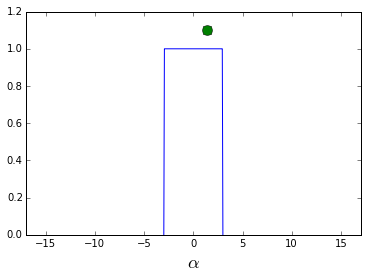## Does the order matter?¶

In :
fig,axs = subplots(2,6,sharex=True)
fig.set_size_inches((10,3))
for n,ax in enumerate(axs.flatten()):
if n==0:
ax.plot(xi,[S.lambdify(x,palpha)(i) for i in xi])
ax.plot(x_samples,1.1,'o',ms=10.)
else:
g = S.prod(map(f,x_samples[:n]))
mxval = S.lambdify(a,g)(xi).max()*1.1
ax.plot(xi,S.lambdify(a,g)(xi))
ax.plot(x_samples[:n],mxval*ones(n),'o',color='gray',alpha=0.3)
ax.plot(x_samples[n],mxval,'o',ms=10.)
ax.axis(ymax=mxval*1.1)
ax.set_yticklabels([''])
ax.tick_params(labelsize=6)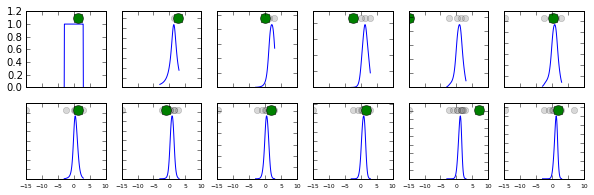In :
# use random  order for first 12 samples
x_samples[:12]= np.random.permutation(x_samples[:12])
fig2,axs = subplots(2,6,sharex=True)
fig2.set_size_inches((10,3))

for n,ax in enumerate(axs.flatten()):
if n==0:
ax.plot(xi,[S.lambdify(x,palpha)(i) for i in xi])
ax.plot(x_samples,1.1,'o',ms=10.)
else:
g = S.prod(map(f,x_samples[:n]))
mxval = S.lambdify(a,g)(xi).max()*1.1
ax.plot(xi,S.lambdify(a,g)(xi))
ax.plot(x_samples[:n],mxval*ones(n),'o',color='gray',alpha=0.3)
ax.plot(x_samples[n],mxval,'o',ms=10.)
ax.axis(ymax=mxval*1.1)
ax.set_yticklabels([''])
ax.tick_params(labelsize=6)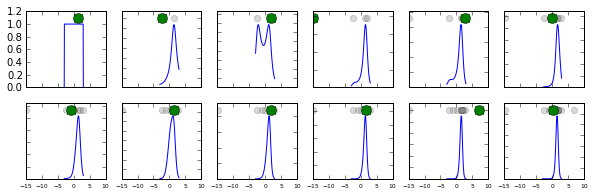In :
fig

Out:## Using Monte Carlo Methods¶

In :
import pymc as pm

WARNING (theano.configdefaults): g++ not detected ! Theano will be unable to execute optimized C-implementations (for both CPU and GPU) and will default to Python implementations. Performance will be severely degraded.

$$f(x \mid \alpha, \eta) = \frac{1}{\pi \beta [1 + (\frac{x-\alpha}{\beta})^2]}$$
In :
with pm.Model() as model:
th = pm.Uniform('th',lower=-pi/2,upper=pi/2)
alpha= pm.Uniform('a',lower=-3,upper=3)
xk = pm.Cauchy('x',beta=1,alpha=alpha,observed=x_samples)
start = pm.find_MAP()
step = pm.NUTS()
trace = pm.sample(300, step, start)

c:\Python27\lib\site-packages\theano-0.6.0-py2.7.egg\theano\gradient.py:512: UserWarning: grad method was asked to compute the gradient with respect to a variable that is not part of the computational graph of the cost, or is used only by a non-differentiable operator: th
handle_disconnected(elem)
c:\Python27\lib\site-packages\theano-0.6.0-py2.7.egg\theano\gradient.py:532: UserWarning: grad method was asked to compute the gradient with respect to a variable that is not part of the computational graph of the cost, or is used only by a non-differentiable operator: <DisconnectedType>
handle_disconnected(rval[i])
c:\Python27\lib\site-packages\theano-0.6.0-py2.7.egg\theano\tensor\subtensor.py:110: FutureWarning: comparison to None will result in an elementwise object comparison in the future.
start in [None, 0] or

 [-----------------100%-----------------] 300 of 300 complete in 424.9 sec
In :
pm.traceplot(trace);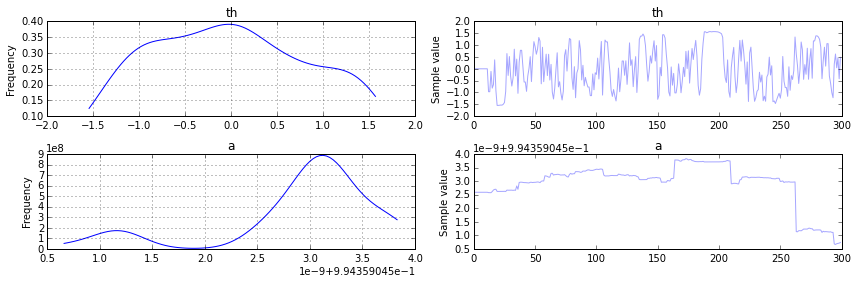In [ ]: